Comparison
Comparison close
Results

CCEM-K4.2017

MEASURAND : Capacitance
NOMINAL VALUE : 10 pF
FREQUENCY : ~ 1.6 kHz

Degrees of equivalence Di and expanded uncertainty Ui (k = 2) in µF/F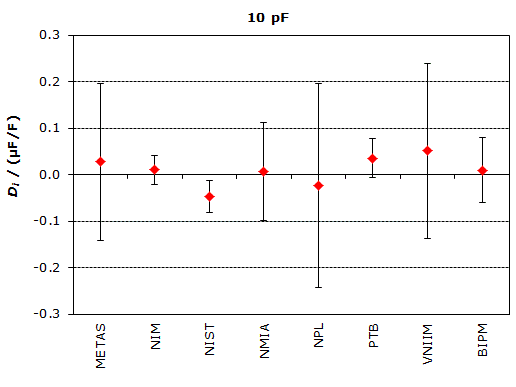CCEM-K4.2017

MEASURAND : Capacitance
NOMINAL VALUE : 100 pF
FREQUENCY : ~ 1.6 kHz

Degrees of equivalence Di and expanded uncertainty Ui (k = 2) in µF/F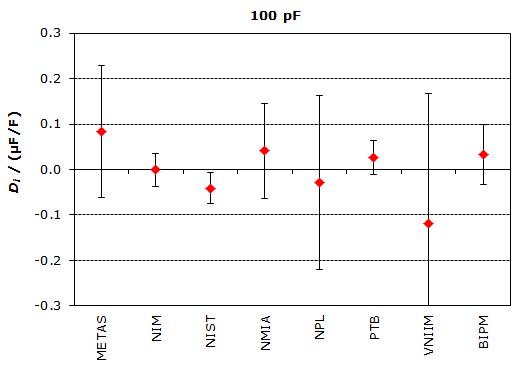CCEM-K4.2017

MEASURAND : Capacitance ratio
NOMINAL VALUE : 100 pF : 10 pF
FREQUENCY : ~ 1.6 kHz

Degrees of equivalence Di and expanded uncertainty Ui (k = 2) in µF/F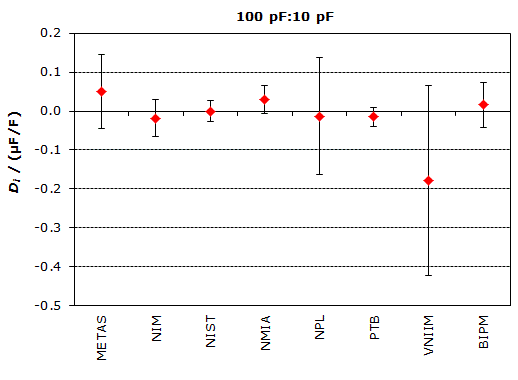Comparison
Comparison close
Results

CCEM-K4.2017

MEASURAND : Capacitance
NOMINAL VALUE : 10 pF
FREQUENCY : ~ 1.6 kHz

Degrees of equivalence Di and expanded uncertainty Ui (k = 2) in µF/F.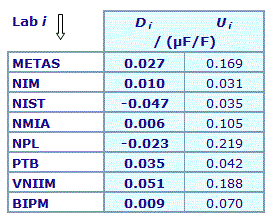Results are presented in A4 format (PDF file).

CCEM-K4.2017

MEASURAND : Capacitance
NOMINAL VALUE : 100 pF
FREQUENCY : ~ 1.6 kHz

Degrees of equivalence Di and expanded uncertainty Ui (k = 2) in µF/F.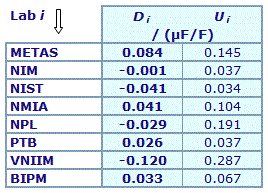Results are presented in A4 format (PDF file).

CCEM-K4.2017

MEASURAND : Capacitance ratio
NOMINAL VALUE : 100 pF : 10 pF
FREQUENCY : ~ 1.6 kHz

Degrees of equivalence Di and expanded uncertainty Ui (k = 2) in µF/F.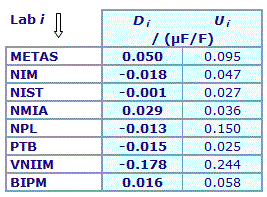Results are presented in A4 format (PDF file).

Comparison
Comparison close
BIPM comparison
 Metrology area, Sub-field Electricity and Magnetism, Capacitance Description Comparison of 10 pF capacitance standards Optional: comparison of 100 pF capacitance standards Time of measurements 2017 Status Approved for equivalence
 Final Reports of the comparisons References Measurand Capacitance at 10 pF (optional capacitance at 100 pF) Parameters Frequency at 1592 Hz (otptional frequency at 1233 Hz) / Voltage 100 V (10 V for optional 100 pF capacitance value) Transfer device The scheme adopted is that of a collapsing star consisting in carrying out simultaneously a large number of bilateral comparisons piloted by the BIPM. The capacitance standards of each of the participant are compared using the BIPM capacitance standards as the common references.
 Comparison type Key Comparison Consultative Committee CCEM (Consultative Committee for Electricity and Magnetism) Conducted by BIPM (Bureau International des Poids et Mesures) Comments This comparison is a repeat of CCEM-K4   Results published on 7 December 2018   Capacitance of nominal value 10 pF Capacitance of nominal value 100 pF Capacitance ratio at 100 pF : 10 pF Pilot institute BIPM Bureau International des Poids et Mesures BIPM - International Organization Contact person P. Gournay +33 (0) 1 45 07 70 07
First Name Last Name
wwww@ww.www +356719836 Institute 1 Institute 1 Khmelnitskiy
Pilot laboratory
BIPM

Bureau International des Poids et Mesures, BIPM - International Organization, N/A

 LNE Laboratoire national de métrologie et d'essais, France, EURAMET
 METAS Federal Institute of Metrology, Switzerland, EURAMET
 NIM National Institute of Metrology, China, APMP
 NIST National Institute of Standards and Technology, United States, SIM
 NMIA National Measurement Institute, Australia, Australia, APMP
 NPL National Physical Laboratory, United Kingdom, EURAMET
 PTB Physikalisch-Technische Bundesanstalt, Germany, EURAMET
 VNIIM D.I. Mendeleyev Institute for Metrology, Rosstandart, Russian Federation, COOMET
Comparison
Comparison close
Results

CCEM-K4.2017

MEASURAND : Capacitance
NOMINAL VALUE : 10 pF
FREQUENCY : ~ 1.6 kHz

 The key comparison reference value KCRV10pF was calculated as the weighted mean of the relative differences Δi from the nominal capacitor value of the laboratories Labi. KCRV10pF = -0.010 × 10-6 with an associated weighted uncertainty  of u10pF = 0.011 × 10-6.

 The degree of equivalence of laboratory i with respect to the reference value is given by a pair of terms, both expressed in µF/F: Di = (Δi - KCRV10pF) and its expanded uncertainty Ui (k = 2) where Ui = 2 (u2(Δi) - u10pF2)1/2.

CCEM-K4.2017

MEASURAND : Capacitance
NOMINAL VALUE : 100 pF
FREQUENCY : ~ 1.6 kHz

 The key comparison reference value KCRV100pF was calculated as the weighted mean of the relative differences Δi from the nominal capacitor value of the laboratories Labi. KCRV100pF = -0.033 × 10-6 with an associated weighted uncertainty  of u100pF = 0.011 × 10-6.

 The degree of equivalence of laboratory i with respect to the reference value is given by a pair of terms, both expressed in µF/F: Di = (Δi - KCRV100pF) and its expanded uncertainty Ui (k = 2) where Ui = 2 (u2(Δi) - u100pF2)1/2.

CCEM-K4.2017

MEASURAND : Capacitance ratio
NOMINAL VALUE : 100 pF : 10 pF
FREQUENCY : ~ 1.6 kHz

 The key comparison reference value KCRV10:1 was calculated as the weighted mean of the difference εi between the capacity ratio Labi and the BIPM. KCRV10:1 = -0.016 × 10-6 with an associated weighted uncertainty  of u10:1 = 0.008 × 10-6.

 The degree of equivalence of laboratory i with respect to the reference value is given by a pair of terms, both expressed in µF/F: Di = (εi - KCRV10:1) and its expanded uncertainty Ui (k = 2) where Ui = 2 (u2(εi) - u10:12)1/2.

Comparison
Comparison close
Results

CCEM-K4.2017

MEASURAND : Capacitance
NOMINAL VALUE : 10 pF
FREQUENCY : ~ 1.6 kHz

The individual measurement results are presented in Section 10 of the CCEM-K4.2017 Final Report.

CCEM-K4.2017

MEASURAND : Capacitance
NOMINAL VALUE : 100 pF
FREQUENCY : ~ 1.6 kHz

The individual measurement results are presented in Section 10 of the CCEM-K4.2017 Final Report.

CCEM-K4.2017

MEASURAND : Capacitance ratio
NOMINAL VALUE : 100 pF : 10 pF
FREQUENCY : ~ 1.6 kHz

The individual measurement results are presented in Section 13 of the CCEM-K4.2017 Final Report.